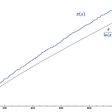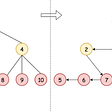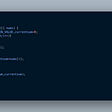# Polynomial Multiplication

Okay, let me start by telling you a story. So, there used to be this 23 yrs. old Russian grad student, Anatoly Karatsuba who used to work under a famous physicist and mathematician — Andrey Kolmogorov

During one of Andrey’s lectures on complexity theory, he stated the limitation on the complexity of performing polynomial multiplication to be bounded by O(n²)

Karatsuba took it as a challenge to do better!

Now, do you realize that the complexity of performing normal polynomial multiplication is O(n²). How?

Let’s say that,

The first polynomial is:
5 + 0x¹ + 10x² + 6x³

And the second polynomial is:
1 + 2x¹ + 4x²

By manual calculation you know that the product polynomial would be:
5 + 10x¹ + 30x² + 26x³ + 52x⁴ + 24x⁵

So, the first polynomial has 4 terms and the second one is having 3, the product would be 4 x 3 = 12 multiplications.

In general, the complexity of polynomial multiplication is then O(m x n) where m and n are the no of terms in the polynomials. If both the polynomials are having equal terms, then the complexity would be, O(n²), obviously.

So, that’s settled. We can shift our focus to the main content here. Story goes on…

Karatsuba comes back after a week with his approach to optimize the calculation so that the overall complexity is reduced. What did he do and how?

Well, forget Karatsuba for a second — there is some very basic arithmetic we are going to do on the polys to develop the approach ourselves. I have done it on a whiteboard already:

Let me explain:

• If you multiply A(x) and B(x) without the optimization as done in the last step, you would end up with 4 terms which is basically the same thing I talked about at the beginning — the naive or elementary approach to multiplication, we have been doing since our elementary math class in school — having the complexity O(n²)

This optimization is precisely what Karatsuba suggested to reduce the total number of unique multiplications that need to be done

Why do the total number of unique multiplications matter?

• We will get to that in a moment…

So, what is all this n/2 thingy here?

This is basically splitting your polynomial into half — having n/2 terms on left and remaining n/2 terms on the right

Okay, how does the formula work?

This is nothing but after splitting the poly into 2 halves, we take x^(n/2) common from the “upper” half and simply rewrite the poly as such.

Okay…why A1, A0, etc.?

Nothing but just denoting the halves — A1 is upper half and A0 is lower half.

Incidentally, writing the poly in this form is part of Karatsuba’s approach. And, rewriting it so have 3 unique multiplications is the approach itself.

That’s it. That’s the theory part. Done.

That was simple…unexpectedly…!

Here is a link to the problem on GFG: https://practice.geeksforgeeks.org/problems/multiply-two-polynomals0721/1

But wait, we are forgetting something. I promised to throw light on 3 multiplications:

Well, this is actually solved in code using divide and conquer approach wherein we use recursion to multiply the 3 terms in the formula and since the problem size just grows smaller and smaller, it happens to be a classic example of divide and conquer strategy, you may find in algorithmic courses.

Well, you have reached till here and quite don’t understand what’s all this about. Here is a video for some context on the problem statement: https://www.youtube.com/watch?v=JCbZayFr9RE

I don’t mind, it happens…all the time.

Now, read the article again(after the context video, things will start getting clearer)

Okay. What now?

Well, let me make you feel secure by providing an implementation of the naive approach with O(n²) complexity:

` // A[] represents coefficients // of first polynomial // B[] represents coefficients // of second polynomial // m and n are sizes of A[] and B[] respectively static int[] multiply(int A[], int B[],       int m, int n) {  int[] prod = new int[m + n - 1];  // Initialize the product polynomial  for (int i = 0; i < m + n - 1; i++){    prod[i] = 0;  }  // Multiply two polynomials term by term  // Take ever term of first polynomial  for (int i = 0; i < m; i++){   // Multiply the current term of first polynomial   // with every term of second polynomial.   for (int j = 0; j < n; j++){    prod[i + j] += A[i] * B[j];   }  }  return prod; }`

Link to GeeksforGeeks for the complete working code: https://www.geeksforgeeks.org/multiply-two-polynomials-2/

The Karatsuba implementation goes something like this:

`private double[] karatsubaMultiplyRecursive(double[] multiplicand, double[] multiplier) {double[] product = new double[2 * multiplicand.length];// Handle the base case where the polynomial has only one                                                                     coefficient                         if (multiplicand.length == 1) {                              product = multiplicand * multiplier;                                   return product;                         }                                                  int halfArraySize = multiplicand.length / 2;                                                 // Declare arrays to hold halved factors  double[] multiplicandLow = new double[halfArraySize];                         double[] multiplicandHigh = new double[halfArraySize];                         double[] multipliplierLow = new double[halfArraySize];                         double[] multipliierHigh = new double[halfArraySize];                                                 double[] multiplicandLowHigh = new double[halfArraySize];                         double[] multipliplierLowHigh = new double[halfArraySize];// Fill in the low and high arraysfor (int halfSizeIndex = 0; halfSizeIndex < halfArraySize; ++halfSizeIndex) {                                                  multiplicandLow[halfSizeIndex] = multiplicand[halfSizeIndex];                                   multiplicandHigh[halfSizeIndex] = multiplicand[halfSizeIndex + halfArraySize];                                multiplicandLowHigh[halfSizeIndex] =           multiplicandLow[halfSizeIndex] +     multiplicandHigh[halfSizeIndex];                                                           multipliplierLow[halfSizeIndex] = multiplier[halfSizeIndex];                              multipliierHigh[halfSizeIndex] = multiplier[halfSizeIndex + halfArraySize];                              multipliplierLowHigh[halfSizeIndex] = multipliplierLow[halfSizeIndex] + multipliierHigh[halfSizeIndex];                                                   }                                                  // Recursively call method on smaller arrays and construct the low and high parts of the productdouble[] productLow = karatsubaMultiplyRecursive(multiplicandLow, multipliplierLow);                         double[] productHigh = karatsubaMultiplyRecursive(multiplicandHigh, multipliierHigh);                                                  double[] productLowHigh = karatsubaMultiplyRecursive(multiplicandLowHigh, multipliplierLowHigh);                                                  //Construct the middle portion of the product                         double[] productMiddle = new double[multiplicand.length];                         for (int halfSizeIndex = 0; halfSizeIndex < multiplicand.length; ++halfSizeIndex) {                              productMiddle[halfSizeIndex] = productLowHigh[halfSizeIndex] -     productLow[halfSizeIndex] - productHigh[halfSizeIndex];                         }                                                  // Assemble the product from the low, middle and high parts. Start with the low and high parts of the product.                         for (int halfSizeIndex = 0, middleOffset = multiplicand.length / 2; halfSizeIndex < multiplicand.length; ++halfSizeIndex) {                              product[halfSizeIndex] += productLow[halfSizeIndex];                              product[halfSizeIndex + multiplicand.length] +=     productHigh[halfSizeIndex];                              product[halfSizeIndex + middleOffset] +=     productMiddle[halfSizeIndex];                         }                                                  return product;                                                 }`

Here is the complete source code for reference:

And, here are some implementation notes:

• For the partitioning of the polynomial, we would try to have equal terms for A1 and A0 but for odd number of terms, have more terms for A1 — Similar partitioning for B
• Normalize: we need to make sure that the degree of both the polynomials is made equal by padding the “shorter” polynomial with 0’s as the coefficients for the higher powers that are missing

Hope that helped. Am open to feedback as this happens to be my second post on medium. I will try to incorporate your feedback in making the post better. So, all comments and suggestions are welcome.

Have a good day!

--

--

--

## More from Manish Sharma

Android Dev | Android Hacker

Love podcasts or audiobooks? Learn on the go with our new app.

## Do the Math## What is The Difference between Student T-Test And Chi-Square Test?## Gosloto 7–49 Morning 10–30 AM Predictions: Wednesday 22 June 2022## Uk49s Lunchtime Predictions: Sunday 19 June 2022## Vectors and Scalars — Linear Algebra for QC## Hyperbolic Floors## QML — Quantum Oracle## Fantastical Primes and Where to Find Them## Manish Sharma

Android Dev | Android Hacker

## Binary Tree Threading & Morris In-order Traversal## 431. Encode N-ary Tree to Binary Tree## Largest Sum Contiguous Sub-Array